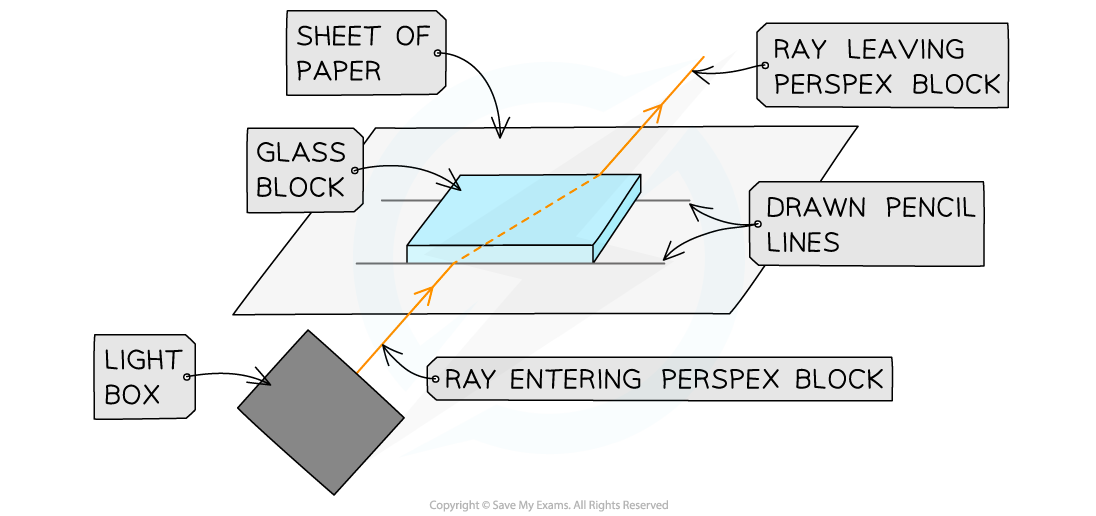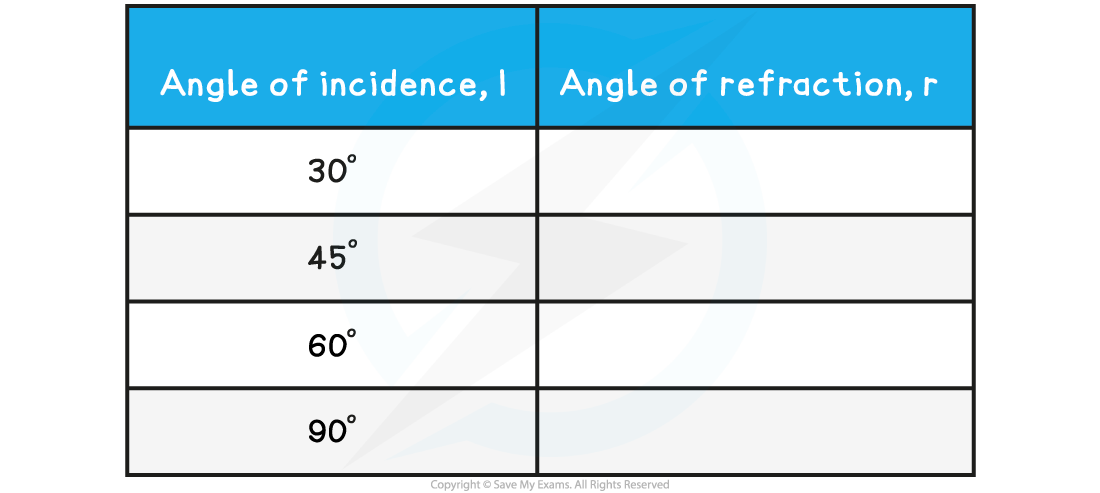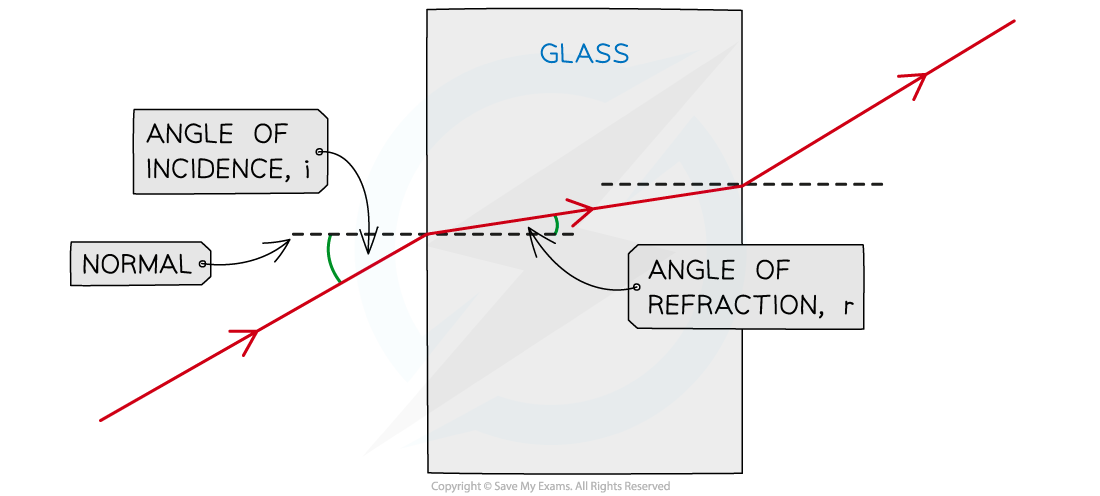# IB DP Physics: SL复习笔记4.4.4 Determining Refractive Index

### Determining Refractive Index

#### Aim of the Experiment

• To investigate the refraction of light by a perspex block

#### Equipment

• Ray Box - to provide a narrow beam of light to refract through the perspex box
• Protractor - to measure the light beam angles
• Sheet of paper - to mark with lines for angle measurement
• Pencil - to make perpendicular line and angle lines on paper
• Ruler - to draw straight lines on the paper
• Perspex block - to refract the light beam
• Resolution of measuring equipment:
• Protractor = 1°
• Ruler = 1 mm

Variables

• Dependent variable = angle of refraction , r
• Control variables:
• Use of the same perspex block
• Width of the light beam
• Same frequency / wavelength of the light

#### MethodApparatus to investigate refraction

1. Place the glass block on a sheet of paper, and carefully draw around the block using a pencil
2. Switch on the ray box and direct a beam of light at the side face of the block
3. Mark on the paper:
• A point on the ray close to the ray box
• The point where the ray enters the block
• The point where the ray exits the block
• A point on the exit light ray which is a distance of about 5 cm away from the block
4. Draw a dashed line normal (at right angles) to the outline of the block where the points are
5. Remove the block and join the points marked with three straight lines
6. Replace the block within its outline and repeat the above process for a ray striking the block at a different angle
• An example of the data collection table is shown below:#### Analysis of Results

• i and r are always measured from the normal
• For light rays entering perspex block, the light ray refracts towards the central line:

i > r

• For light rays exiting the perspex block, the light ray refracts away from the central line:

i < r

• When the angle of incidence is 90° to the perspex block, the light ray does not refract, it passes straight through the block:

i = r

• If the experiment was carried out correctly, the angles should follow the pattern, as shown below:How to measure the angle of incidence and angle of refraction

#### Evaluating the Experiment

Systematic Errors:

• An error could occur if the 90° lines are drawn incorrectly
• Use a set square to draw perpendicular lines
• If the mirror is distorted, this could affect the reflection angle, so make sure there are little to no blemishes on it

Random Errors:

• The points for the incoming and reflected beam may be inaccurately marked
• Use a sharpened pencil and mark in the middle of the beam
• The protractor resolution may make it difficult to read the angles accurately
• Use a protractor with a higher resolution

#### Safety Considerations

• The ray box light could cause burns if touched
• Run burns under cold running water for at least five minute
• Looking directly into the light may damage the eyes
• Avoid looking directly at the light
• Stand behind the ray box during the experiment
• Keep all liquids away from the electrical equipment and paper